﻿ 基于Motif的社交网络用户影响力排序方法研究

# 基于Motif的社交网络用户影响力排序方法研究Research on Social Network User Impact Ranking Method Based on Motif

Abstract: LeaderRank has been widely used in ranking algorithms to measure the influence or importance of users in social networks, but traditional LeaderRank only uses edge-based relationships and ignores high-order relationships captured by a subgraph composed of a small number of nodes. In this paper, we propose a Motif-based LeaderRank (MLR) algorithm, which incorporates the higher-order relationships of Motif into the LeaderRank algorithm to improve the ranking effect of social network user influence. We perform experiments on the Twitter dataset, and the results not only show the feasibility of the MLR algorithm, but also significantly improve the accuracy of the ranking of user influence in social networks. In addition to comparing with the baseline algorithm, the parameters of the MLR algorithm are also analyzed, which shows that the MLR algorithm is better than LeaderRank when ranking user influence in social networks.

1. 引言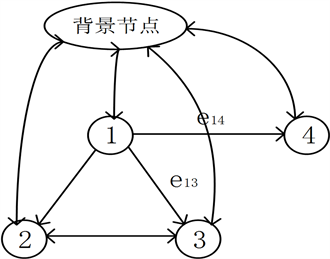Figure 1. A schematic diagram of the LeaderRank algorithm. The algorithm introduces a background node in the original networkFigure 2. 3-Node simple Motif based on social network triangle relationship in directed unweighted graph

2. 相关研究

2.2. 复杂网络中Motif的识别问题

3.1. 问题描述

$x={P}^{\text{T}}\cdot x$ (1)

${\text{MLR}}_{i}={x}_{i}+\frac{{x}_{n+1}}{N}$ (2)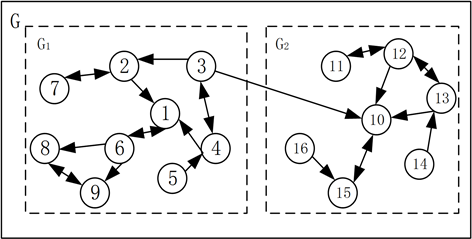Figure 3. Network without attributes

3.2. Motif定义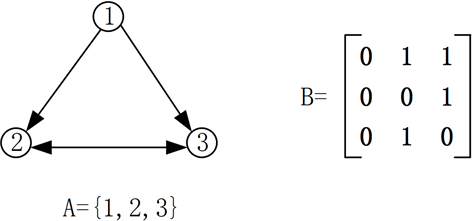Figure 4. An example for a simple 3-nodes Motif

$F\left(B,A\right)=\left\{\left(set\left(v\right),set\left(\delta A\left(v\right)\right)\right)|v\in {V}^{k},{v}_{1},\cdots ,{v}_{k},distinct,{W}_{v}=B\right\}$ (3)

$F\left(B,A\right)=\left\{\left(\left\{1,2,3\right\},\left\{1,2\right\}\right),\left(\left\{1,2,5\right\},\left\{1,2\right\}\right)\right\}$ (4)

3.3. 基于Motif的邻接矩阵

${\left({W}_{M}\right)}_{ij}=\underset{\left(v,\delta A\left(v\right)\right)\in F}{\sum }l\left(\left\{i,j\right\}\subset \delta A\left(v\right)\right)$ (5)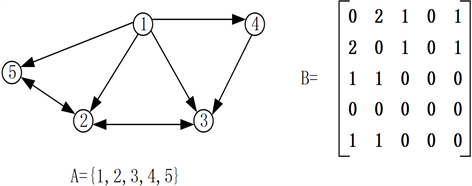Figure 5. An example for computing M6 based adjacency matrix

${W}_{{M}_{6}}=\left(U\cdot B\right)\Theta U+\left(B\cdot {U}^{\text{T}}\right)\Theta {U}^{\text{T}}+\left({U}^{\text{T}}\cdot U\right)\Theta B$ (6)

3.4. 基于M6邻接矩阵的案例

1) 社区发现算法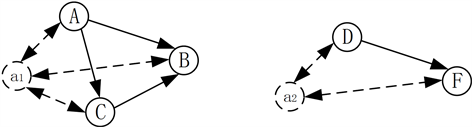Figure 6. Virtual node

$Affect\left({A}_{i}\right)=\frac{\underset{i}{\sum }\underset{j}{\sum }{\left({W}^{Att{r}^{2}}\right)}_{ij}-\underset{i}{\sum }\underset{j}{\sum }{\left({{W}^{\prime }}^{2}\right)}_{ij}}{{R}^{\beta A}}$ (7)

$strengt{h}_{ij}={s}_{ij}^{out}+{s}_{ij}^{in}+{s}_{ij}^{Attr}$ (8)

${s}_{ij}^{out}=\left\{\begin{array}{l}0,{{w}^{\prime }}_{ij}=0\\ 1+{e}^{1-\beta out\underset{h}{\sum }{w}_{ih}},{{w}^{\prime }}_{ij}=1\end{array}$ (9)

${s}_{ij}^{in}=\left\{\begin{array}{l}0,{{w}^{\prime }}_{ji}=0\\ e/{2}^{1-\beta in\underset{h}{\sum }{w}_{hi}},{{w}^{\prime }}_{ji}=1\end{array}$ (10)

${s}_{ij}^{Attr}=\left\{\begin{array}{l}0,{y}_{ij}=0\\ \frac{1-{1.1}^{1-\beta Attr\underset{h}{\sum }{w}_{ih}}}{2/\left(1+\mathrm{log}{D}^{\prime }\right)},{y}_{ij}=1\end{array}$ (11)

${{P}^{\prime }}_{ij}=\frac{{s}_{ij}}{\underset{k}{\sum }{s}_{ih}}$ (12)

2) 基于Motif的邻接矩阵Figure 7. Community G1 (16,17) subgraph structure diagram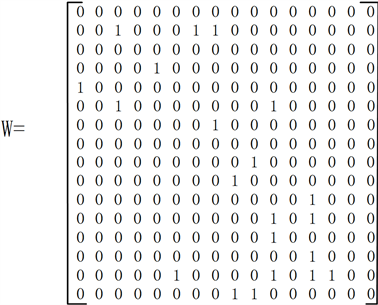Figure 8. Community G1 (16,17) first-order matrix W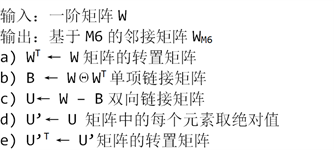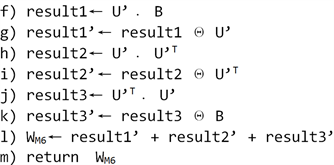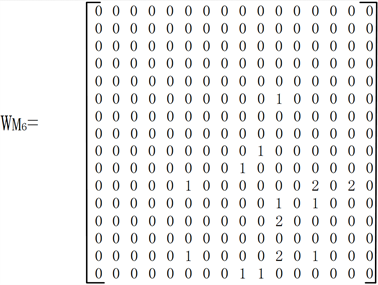Figure 9. Community G1 (16,17) based on the M6 adjacency matrix ${W}_{{\text{M}}_{6}}$

${H}_{{\text{M}}_{k}}=\alpha \cdot W+\left(1-\alpha \right)\cdot {W}_{{\text{M}}_{k}}$ (13)

${\left({P}_{{\text{M}}_{k}}\right)}_{ij}={\left({H}_{{\text{M}}_{k}}\right)}_{ij}/\underset{i}{\sum }{\left({H}_{{\text{M}}_{k}}\right)}_{ij}$ (14)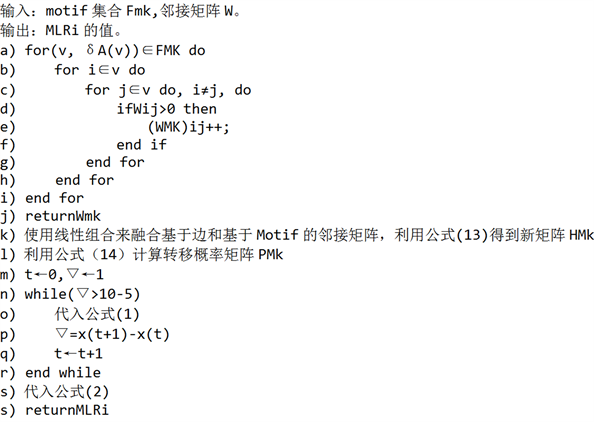4. 实验

4.1. 实验数据集

4.2. 实验环境

4.3. 评价指标

NDCG计算公式表示为

$\text{NDCG}@\text{N}=\frac{\text{DCG}@\text{N}}{\text{IDCG}@\text{N}}$ (15)

$\text{DCG}@\text{N}=\underset{i=1}{\overset{N}{\sum }}\frac{{2}^{re{l}_{i}}-1}{{\mathrm{log}}_{2}\left(i+1\right)}$ (16)

$\text{IDCG}@\text{N}=\underset{i=1}{\overset{|REL|}{\sum }}\frac{{2}^{re{l}_{i}}-1}{{\mathrm{log}}_{2}\left(i+1\right)}$ (17)

4.4. 实验结果分析

1) 可行性与准确性分析Table 1. NDCG for top10, top50, top200 users from Twitter

2) α值分析Table 2. NDCG values of M1~M7 in Twitter dataset top10, top50, top200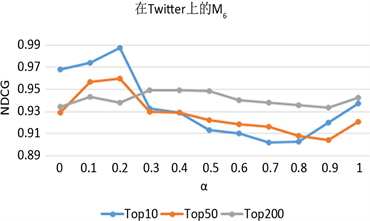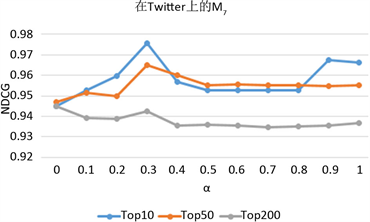(a)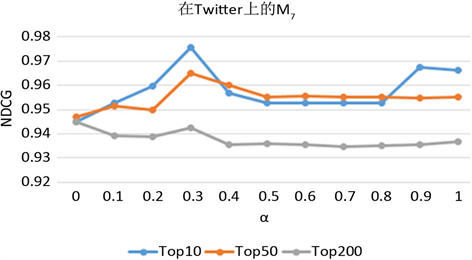(b)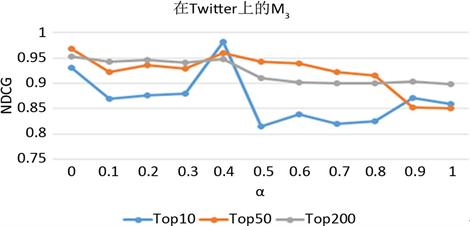(c)

Figure 10. Parameter analysis of α on the three datasets. (a) Running alpha parameter analysis of M6 on Twitter; (b) Running alpha parameter analysis of M7 on Twitter; (c) Running alpha parameter analysis of M3 on Twitter

3) 案例分析Table 3. The average of the NDCG values of the top 10 users

5. 结束语

 Song, X., Chi, Y., Hino, K., et al. (2007) Identifying Opinion Leaders in the Blogosphere. Proceedings of the 16th ACM Conference on Information and Knowledge Management, CIKM 2007, Lisbon, 6-10 November 2007, 971-974.
https://doi.org/10.1145/1321440.1321588

 Tang, J., Sun, J., Wang, C., et al. (2009) Social Influence Analysis in Large-Scale Networks. Proceedings of the 15th ACM SIGKDD International Conference on Knowledge Discovery and Data Mining, Paris, 28 June-1 July 2009, 807-816.
https://doi.org/10.1145/1557019.1557108

 Xiang, B., Liu, Q., Chen, E., et al. (2013) PageRank with Priors: An Influence Propagation Perspective. Proceedings of the 23rd International Joint Conference on Artificial Intelligence, AAAI Press, Palo Alto, 2740-2746.

 Wang, Y., Wang, X., Tang, J., et al. (2015) Modeling Status Theory in Trust Prediction. AAAI, 1875-1881.

 Page, L., Brin, S., Motwani, R. and Winograd, T. (1999) The Pagerank Citation Ranking: Bringing Order to the Web. Proceedings of the 7th Interna-tional World Wide Web Conference, Brisbane, 1998, 161-172.

 Lü, L.Y., Zhang, Y.-C., Yeung, C.H. and Zhou, T. (2011) Leaders in Social Networks, the Delicious Case. PLoS ONE, 6, e21202.
https://doi.org/10.1371/journal.pone.0021202

 Jiang, X., Sun, X. and Zhuge, H. (2013) Graph-Based Algo-rithms for Ranking Researchers: Not All Swans Are White! Scientometrics, 96, 743-759.
https://doi.org/10.1007/s11192-012-0943-y

 邓启平, 王小梅. 利用LeaderRank识别有影响力的作者[J]. 现代图书情报技术, 2015, 31(9): 60-67.

 徐郡明, 朱福喜, 刘世超, 等. 改进LeaderRank算法的意见领袖挖掘[J]. 计算机工程与应用, 2015(1): 110-114.

 Zhang, Z.H., Jiang, G.P., Song, Y.R., et al. (2017) An Improved Weighted LeaderRank Algorithm for Identifying Influential Spreaders in Complex Networks. An Improved Weighted LeaderRank Algorithm for Identifying Influential Spreaders in Complex Networks. IEEE International Conference on Computational Science and Engineering (CSE) and IEEE International Conference on Embedded and Ubiquitous Com-puting (EUC), Guangzhou, 21-24 July 2017.
https://doi.org/10.1109/CSE-EUC.2017.146

 Bakshy, E., Hofman, J.M., Mason, W.A., et al. (2015) Every-one’s an Influencer: Quantifying Influence on Twitter. Proceedings of the 4th ACM International Conference on Web Search and Data Mining, Hong Kong, 9-12 February 2011.

 顾亦然, 朱梓嫣. 基于LeaderRank和节点相似度的复杂网络重要节点排序算法[J]. 电子科技大学学报, 2017, 46(2): 441-448.

 Watts, D.J. and Strogatz, S.H. (1998) Collective Dynamics of Small World Networks. Nature, 393, 440-442.
https://doi.org/10.1038/30918

 Sen, P., Dasgupta, S., Chatterjee, A., et al. (2003) Small-World Properties of the Indian Railway Network. Physical Review E, 67, 036106.
https://doi.org/10.1103/PhysRevE.67.036106

 Barabási, A.-L. and Albert, R. (1999) Emergence of Scaling in Random Networks. Science, 286, 509-512.
https://doi.org/10.1126/science.286.5439.509

 Barabási, A.-L., Albert, R. and Jeong, H. (2000) Scale-Free Characteristics of Random Networks: The Topology of the World-Wide Web. Physica A, 281, 69-77.
https://doi.org/10.1016/S0378-4371(00)00018-2

 Milo, R., Shen-Orr, S., Itzkovitz, S., et al. (2002) Network Motifs: Simple Building Blocks of Complex Networks. Science, 298, 824-827.
https://doi.org/10.1126/science.298.5594.824

 Ugander, J., Backstrom, L. and Kleinberg, J.M. (2013) Sub-graph Frequencies: Mapping the Empirical and Extremal Geography of Large Graph Collections. Proceedings of the 22nd international conference on World Wide Web, Rio de Janeiro, May 2013, 1307-1318.
https://doi.org/10.1145/2488388.2488502

 Rotabi, R., Kamath, K., Kleinberg, J., et al. (2017) Detecting Strong Ties Using Network Motifs. Proceedings of the 26th International Conference on World Wide Web, Perth, 3-7 April 2017, 983-992.
https://doi.org/10.1145/3041021.3055139

 Wang, P., Lü, J.H. and Yu, X. (2014) Identification of Important Nodes in Directed Biological Networks: A Network Motif Approach. PLoS ONE, 9, e106132.
https://doi.org/10.1371/journal.pone.0106132

 Nataša, P. (2007) Biological Network Comparison Using Graphlet Degree Distribution. Bioinformatics, 23, e177-e183.

 Ahmed, N.K., Neville, J., Rossi, R.A., et al. (2015) Efficient Graphlet Counting for Large Networks. 2015 IEEE International Conference on Data Mining (ICDM). 2015 IEEE International Conference on Data Mining, Atlantic City, 14-17 November 2015.
https://doi.org/10.1109/ICDM.2015.141

 Jha, M., Seshadhri, C. and Pinar, A. (2014) Path Sampling: A Fast and Provable Method for Estimating 4-Vertex Subgraph Counts. Proceedings of the 24th International Conference on World Wide Web, Florence, 18-22 May 2015, 495-505.
https://doi.org/10.1145/2736277.2741101

 Han, G. and Sethu, H. (2016) Waddling Random Walk: Fast and Accurate Mining of Motif Statistics in Large Graphs. 2016 IEEE 16th International Conference on Data Mining (ICDM), Barcelona, 12-15 December 2016.
https://doi.org/10.1109/ICDM.2016.0029

 Benson, A.R., Gleich, D.F. and Leskovec, J. (2016) Higher-Order Organization of Complex Networks. Science, 353, 163-166.